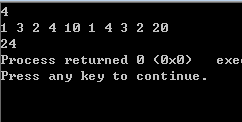# 动态规划-数字三角形问题

1

3 2

4 10 1

4 3 2 20d(i,j) = a(i,j) + max{d(i+1,j),d(i+1,j+1)}

int a,n,d;

int solve1(int i,int j)
{
/* 递归 (重复计算，效率低) O(2^n)
把当前位置(i,j)看成一个状态 d[i][j]为从格子出发能得到的最大和 解为d
d(i,j)=a(i,j) +max {d(i+1,j),d(i+1,j+1)} */
return a[i][j]+(i==n? 0 : max(solve1(i+1,j),solve1(i+1,j+1)));
}

int solve2()
{
/*递推 (逆序枚举) O(n^2)
i是逆序枚举的，计算d[i][j]前 所需要的d[i+1][j]
和d[i+1][j+1]一定计算出来了*/
int i,j;
for(j=1;j<=n;j++)
d[n][j]=a[n][j];
for(i=n-1;i>=1;i--)
for(j=1;j<=i;j++)
d[i][j]=a[i][j]+max(d[i+1][j],d[i+1][j+1]);
return d;
}

int solve3(int i,int j)
{
//记忆化搜索 O(n^2)
//题目中所说的各个数都是非负的 因此如果计算过某个d[i][j] 则应该非负
if(d[i][j]>=0) //已经计算过
return d[i][j];
return d[i][j]=a[i][j]+(i==n? 0 : max(solve1(i+1,j),solve1(i+1,j+1)));
}

12-1902-21
12-22
10-155898
05-291万+
01-266732
03-182541
10-276832
©️2020 CSDN 皮肤主题: 书香水墨 设计师:CSDN官方博客